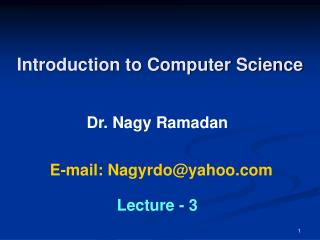DownloadDownload PresentationIntroduction to Computer Science

# Introduction to Computer Science

Download Presentation## Introduction to Computer Science

- - - - - - - - - - - - - - - - - - - - - - - - - - - E N D - - - - - - - - - - - - - - - - - - - - - - - - - - -
##### Presentation Transcript

1. Introduction to Computer Science Dr. Nagy Ramadan E-mail: Nagyrdo@yahoo.com Lecture - 3

2. Outline • Part 1 - Conversions in Numbering Systems • Part 2 - Conversion from Decimal to Binary System • Part 3 - Conversion from Decimal to Ternary System • Part 4 - Conversion from Decimal to Octal System • Part 5 - Conversion from Decimal to Hexadecimal System • Part 6 - Conversion from Binary to Octal System and vice versa • Part 7 - Conversion from Binary to Hexadecimal System and vice versa

3. Conversions in Numbering Systems In lecture (2), we have studied the conversion from any number system to the decimal one, i.e. In this lecture, we are going to study the conversions in the reverse direction, i.e.

4. R L L R Conversion from Decimal to Binary System Ex1: Convert the decimal number 174.390625 to its equivalent binary number Therefore, the binary number (10101110.011001)2 is exact equivalent to the decimal number (174.390625)10

5. R L L R Ex2: Obtain the equivalent binary number of the decimal number 47.763 with a precision of 7-binary digits Therefore, the number (10111.1100001)2 is the approximate binary equivalent to the decimal number (47.763)10 with a precision of 7-binary digits .

6. R L L R Conversion from Decimal to Ternary System Ex1: Convert the decimal number 124.33 to its equivalent ternary number with precision of 5 ternary digits Therefore, the number (11121.02222)3 is the approximate ternary equivalent to the decimal number (124.33)10 with a precision of 5-ternary digits

7. R L L R Ex2: Obtain the ternary equivalent of the decimal number Therefore, the number (1110.001)3 is the exact ternary equivalent to the decimal number

8. R L L R Conversion from Decimal to Octal System Ex1: Convert the decimal number 167.390625 to its equivalent octal number Therefore, the number (247.31)8 is the exact octal equivalent to the decimal number (167.390625)10

9. R L L R Ex2: Find the equivalent octal number of the decimal number 95.236 with a precision up to 4 octal digits. Therefore, the number (137.1706)8 is the approximate octal equivalent to the decimal number (95.236)10 with a precision of 4-octal digits

10. R L L R Conversion from Decimal to Hexadecimal System Ex1: Convert the decimal number 247.390625 to its equivalent hexadecimal number Therefore, the number (F7.64)16 is the exact hexadecimal equivalent to the decimal number (247.390625)10

11. Conversion from Binary to Octal System and vice versa 1. Indirect conversion By using the decimal system as the intermediate number system, i.e. B  D  O O D  B 2. Direct Conversion Each 3-binary digits are replaced by one octal digit , and vice versa , using the following table: Octal Binary

12. R L L R Ex1: Convert the binary number 1001101.1011 to octal First Solution (indirect method) Convert the binary number to decimal, then convert the decimal to octal. (1001101.1011)2 = 1*26 +0*25 + 0*24 +1*23 + 1*22 + 0*21 + 1*20 + 1*2-1 + 0*2-2 + 1*2-3 + 1*2-4 = 64 + 8 + 4 + 1 + 0.5 + 0.125 + 0.0625 = (77.6875)10 Thus, (1001101.1011)2 = (77.6875)10 = (115.54)8

13. Second Solution (direct method) Start from the binary point and move to the right and to the left grouping each 3-binary digits as one octal digit. Adding 0’s to the extreme right (or left) is allowed. Thus, (1001101.1011)2 = (115.54)8

14. Ex2: Convert the octal number 115.54 to binary Solution (direct method) 1 1 5 . 5 4 001 001 101 . 101 100 Thus, (115.54)8 = (001001101.101100)2 or (115.54)8 = (1001101.1011)2

15. Conversion from Binary to Hexadecimal System and vice versa 1. Indirect conversion By using the decimal system as the intermediate number system, i.e. B  D  H H D  B 2. Direct Conversion Each 4-binary digits are replaced by one hexadecimal digit, and vice versa, Using the following table: Hexadecimal Binary

16. Ex: Convert the hexadecimal number 3BC. 2E directly to its equivalent binary number. Solution Thus, (3BC.2E)16 = (001110111100.00101110)2

17. Ex: Convert the binary number 111010.11011 directly to its equivalent hexadecimal number Solution Start from the binary point and move to the right and to the left grouping each 4- binary digits as one hexadecimal digit. Adding 0’s to the extreme right (or left) is allowed. Thus, we have: (111010.11011)2 =(00111010.11011000)2 =( 3 A . D 8 )16

18. Questions